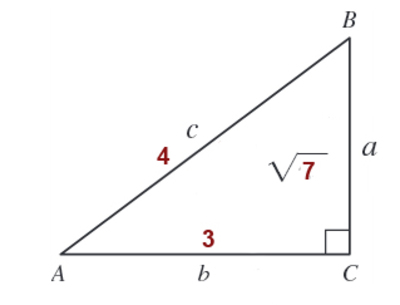## Trigonometry 7th Edition

$\sin A = \frac{\sqrt 7}{4}$ , $\\csc A = \frac{4}{\sqrt 7}$ $\\cos A = \frac{3}{4}$ , $\\sec A = \frac{4}{3}$ $\\tan A = \frac{\sqrt 7}{3}$ , $\\\cot A = \frac{3}{\sqrt 7}$
Steps to Answer- First we will draw the diagram of triangle ABC and then use the given information and Pythagoras Theorem to solve for 'a'- We know that - $c^{2} =a^{2} + b^{2}$ ( Pythagoras Theorem) Therefore - $a^{2} =c^{2} - b^{2}$ $a^{2} = 4^{2} - 3^{2}$ $a^{2} = 16 - 9$ $a^{2} = 7$ therefore $a = \sqrt 7$ Now we can write the required six T-functions of A using $a= \sqrt 7$ , b = 3 and c = 4 $\sin A = \frac{a}{c} = \frac{\sqrt 7}{4}$ $\\csc A = \frac{c}{a} =\frac{4}{\sqrt 7}$ $\\cos A = \frac{b}{c} = \frac{3}{4}$ $\\sec A = \frac{c}{b} = \frac{4}{3}$ $\\tan A = \frac{a}{b} =\frac{\sqrt 7}{3}$ $\\\cot A = \frac{b}{a} = \frac{3}{\sqrt 7}$